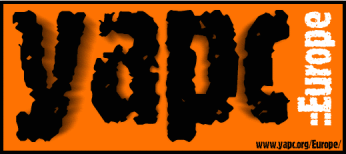Yet Another Perl Conference Europe 2001 August 2-4, 2001 Hogeschool Holland Amsterdam, NetherlandsObfuscated tshirt

The obfuscated Perl code below is the design for the alternative yapc::Europe tshirt. If the code isn't quite clear enough, Philippe explained it during his lightning talk on Friday and his slides are on the proceedings CD.

```#!/usr/bin/perl -wT
use strict;use Safe;(my\$s=new Safe)->permit(qw/rand sort entereval/);@_{s=>'t'
}=(<<Orange,"teozltro--hqaftsy ntqdsiqcg ;-)\r");%_=(%_,split(/(\n^.{24})/m,q[
____    ____   ____     _________ ________                          _
\   \  /   /  /    \   |         V        \ Hogeschool Holland     | |  __  __
\   \/   /  /      \  |     __   \   ____/ __   __   Amsterdam   / __) \ \/ /
\      /  /   /\   \ |    |  \  |  |     /  \ /  \ Netherlands  \__ \  >  <
|    |  /   /__\   \|    |__/  /  |     \__/ \__/ August 2-4   (   / /_/\_\
__|____|_/   _____    \     ____/   |____  __   __     2001      _|_|  __  __
|         \  /     \    \   |    |        \/  \ /  \             / __ \ \ \/ /
|   ______/_/    __ \____\__|____\________/\__/_\__/    ----    / / _` | >  <
|       \ |  |  |  |  V   V  __  \ |   ___ \/  __ \             \ \__,_|/_/\_\
|    ___/ |  |  |  |   ___/ /  \  \|  |___) ) /__) )  Coded by   \___/_ __  __
|   |_____|  |__|  |  | |  |    |  |   ____/  ____/   Philippe   (_)/ / \ \/ /
|         \        |  |  \  \__/  /|  |    \      \    "BooK"      / /_  )  (
|_________/\____,__|__|   \______/ |__|     \_____/    Bruhat     /_/(_)/_/\_\
Orange
____   ___   ___  _  ..(substr(\$n[&f],&g,1)=chr 97+(ord(substr\$n[&f],&g,1)+
(___ \ / _ \_/ _ \/ | \$R='( +)';s/(key)|eval/\$1?"value":""/eg;*n=[split/(\W+
__) | | | | | | | | r())%26)}sub g{\$X%length\$n[&f]}sub f{\$x%3*2}print"\n";
__/ __/| | | | | | | | ){print@n if(select(\$/,\$,,\$<,.1*rand)||(\$X+=\$x+++&r)).
(__)/___| |_| | |_| | | )/,\$_{t}];(\$_=eval)=~y/\n//d;sub r{length};for(split\$R
(_____(_)___(_)___/|_| udy]),'');\$|++;\$s->reval(\$_='eval join"",sort+keys%_')
```